Скачать презентацию Conservation Doing without and more with less

f623601e15c8a13024473a3a94405f78.ppt

• Количество слайдов: 11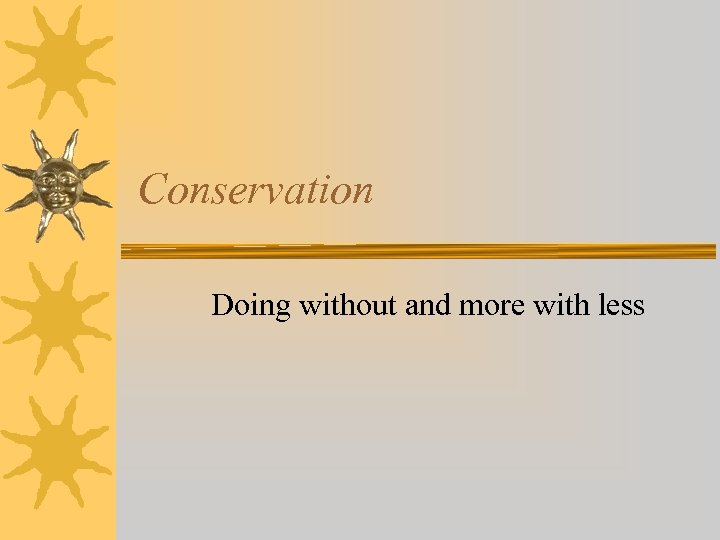Conservation Doing without and more with less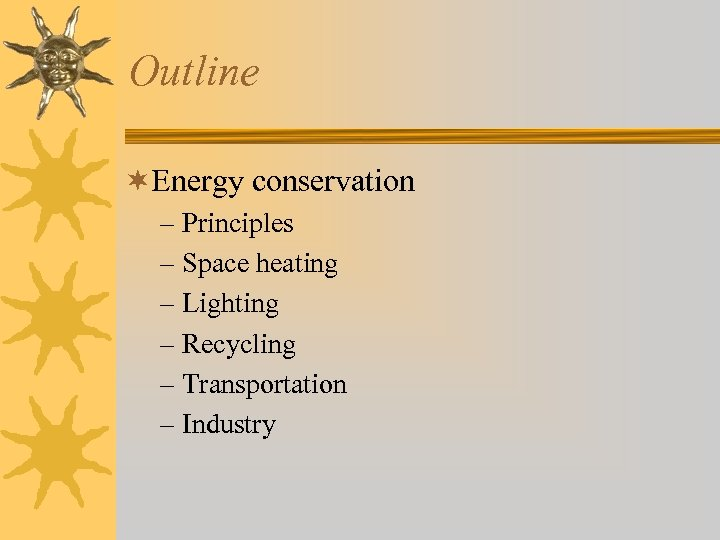Outline ¬Energy conservation – Principles – Space heating – Lighting – Recycling – Transportation – Industry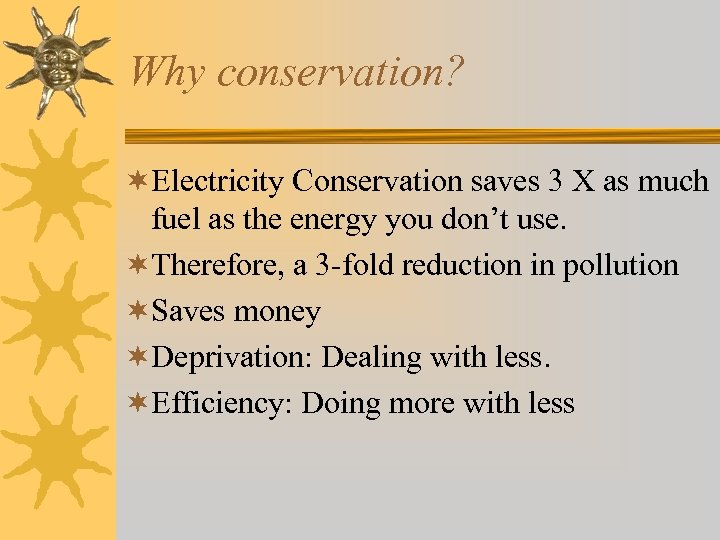Why conservation? ¬Electricity Conservation saves 3 X as much fuel as the energy you don’t use. ¬Therefore, a 3 -fold reduction in pollution ¬Saves money ¬Deprivation: Dealing with less. ¬Efficiency: Doing more with less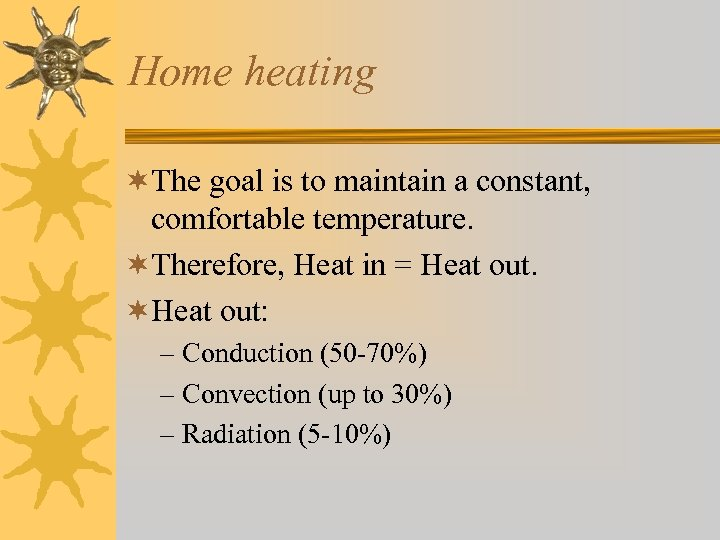Home heating ¬The goal is to maintain a constant, comfortable temperature. ¬Therefore, Heat in = Heat out. ¬Heat out: – Conduction (50 -70%) – Convection (up to 30%) – Radiation (5 -10%)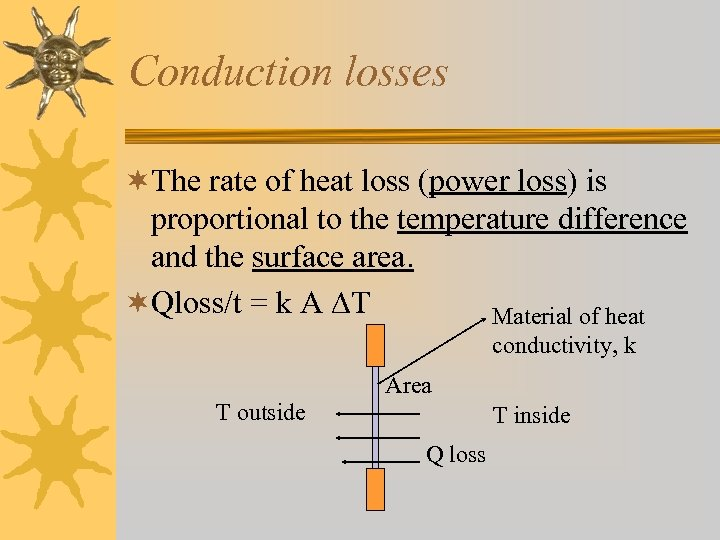Conduction losses ¬The rate of heat loss (power loss) is proportional to the temperature difference and the surface area. ¬Qloss/t = k A T Material of heat conductivity, k Area T outside T inside Q loss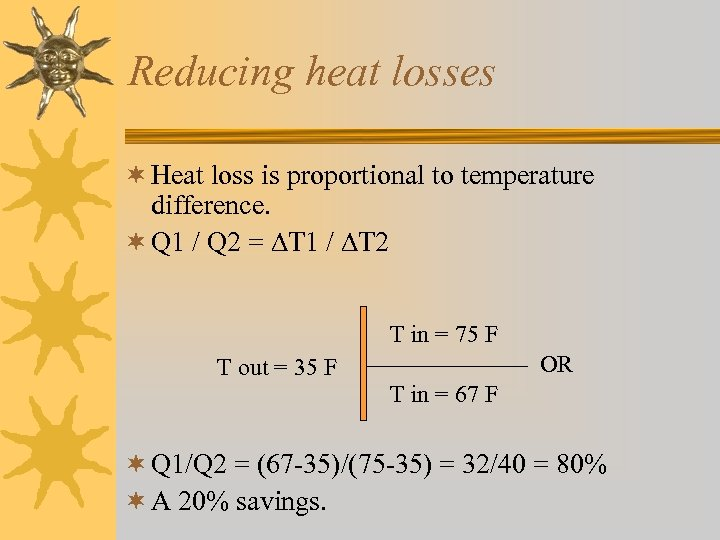Reducing heat losses ¬ Heat loss is proportional to temperature difference. ¬ Q 1 / Q 2 = T 1 / T 2 T in = 75 F OR T out = 35 F T in = 67 F ¬ Q 1/Q 2 = (67 -35)/(75 -35) = 32/40 = 80% ¬ A 20% savings.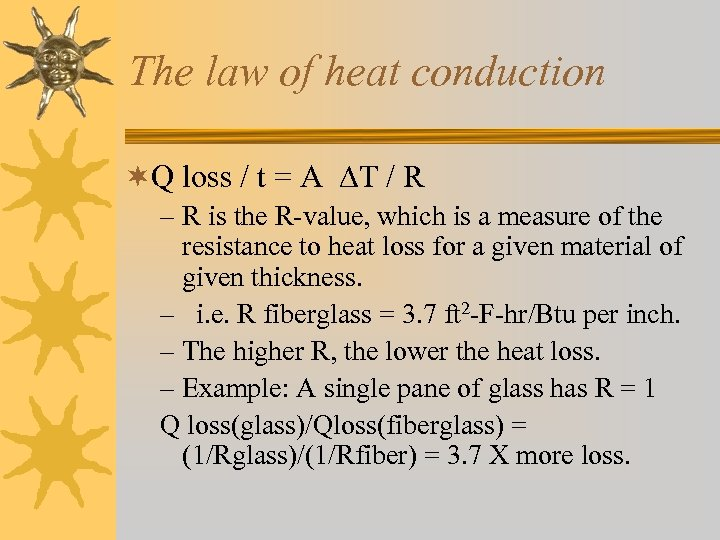The law of heat conduction ¬Q loss / t = A T / R – R is the R-value, which is a measure of the resistance to heat loss for a given material of given thickness. – i. e. R fiberglass = 3. 7 ft 2 -F-hr/Btu per inch. – The higher R, the lower the heat loss. – Example: A single pane of glass has R = 1 Q loss(glass)/Qloss(fiberglass) = (1/Rglass)/(1/Rfiber) = 3. 7 X more loss.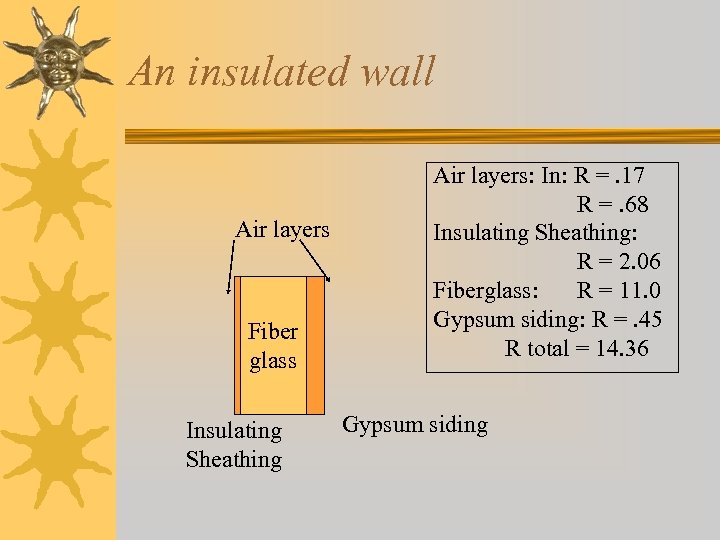An insulated wall Air layers Fiber glass Insulating Sheathing Air layers: In: R =. 17 R =. 68 Insulating Sheathing: R = 2. 06 Fiberglass: R = 11. 0 Gypsum siding: R =. 45 R total = 14. 36 Gypsum siding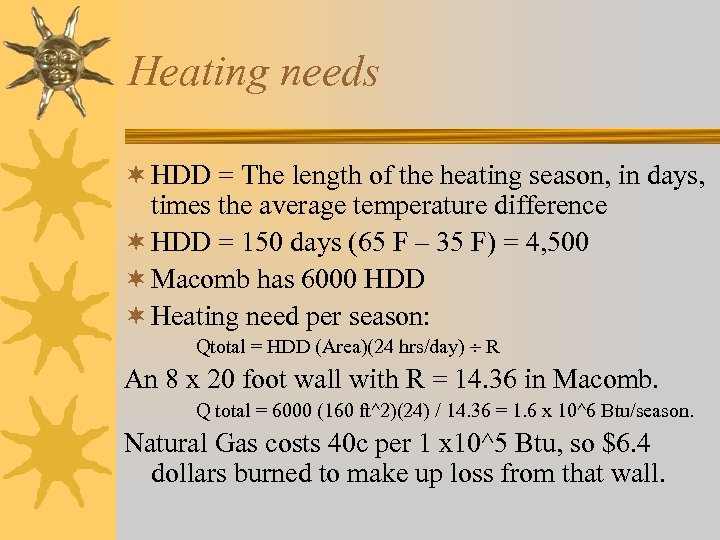Heating needs ¬ HDD = The length of the heating season, in days, times the average temperature difference ¬ HDD = 150 days (65 F – 35 F) = 4, 500 ¬ Macomb has 6000 HDD ¬ Heating need per season: Qtotal = HDD (Area)(24 hrs/day) R An 8 x 20 foot wall with R = 14. 36 in Macomb. Q total = 6000 (160 ft^2)(24) / 14. 36 = 1. 6 x 10^6 Btu/season. Natural Gas costs 40 c per 1 x 10^5 Btu, so \$6. 4 dollars burned to make up loss from that wall.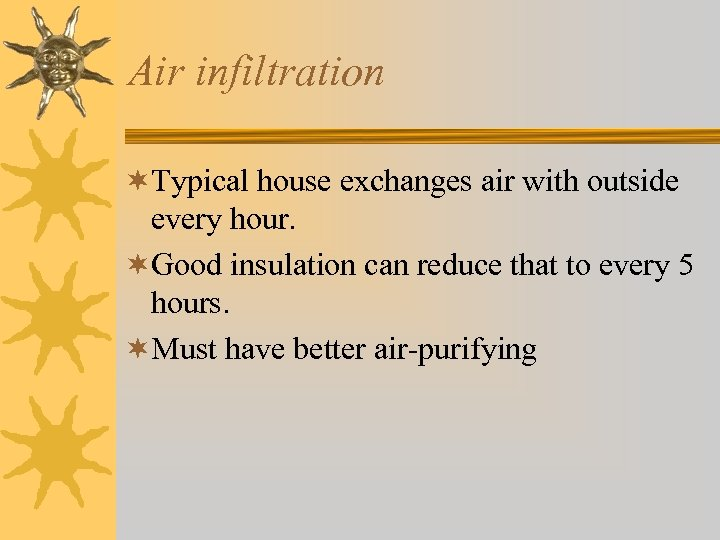Air infiltration ¬Typical house exchanges air with outside every hour. ¬Good insulation can reduce that to every 5 hours. ¬Must have better air-purifying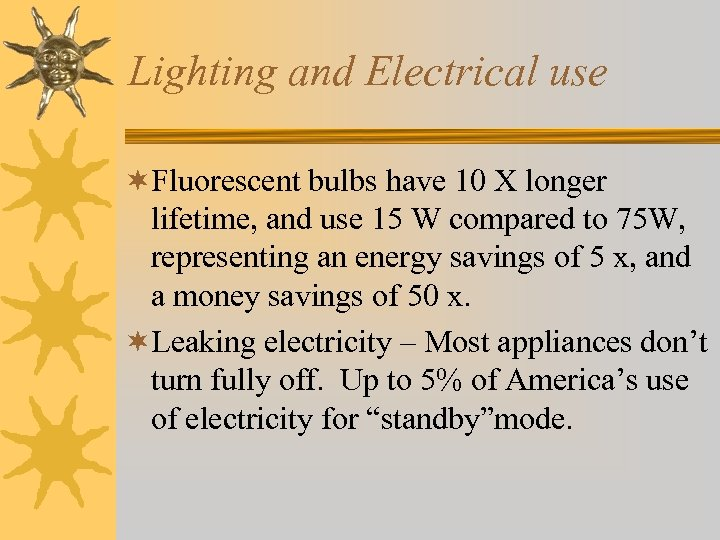Lighting and Electrical use ¬Fluorescent bulbs have 10 X longer lifetime, and use 15 W compared to 75 W, representing an energy savings of 5 x, and a money savings of 50 x. ¬Leaking electricity – Most appliances don’t turn fully off. Up to 5% of America’s use of electricity for “standby”mode.